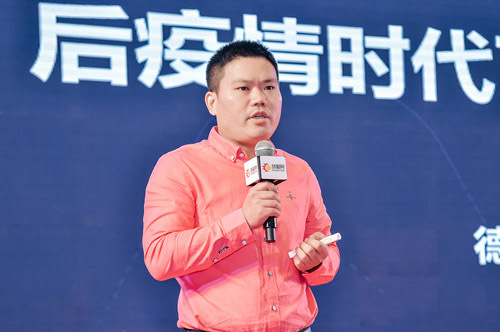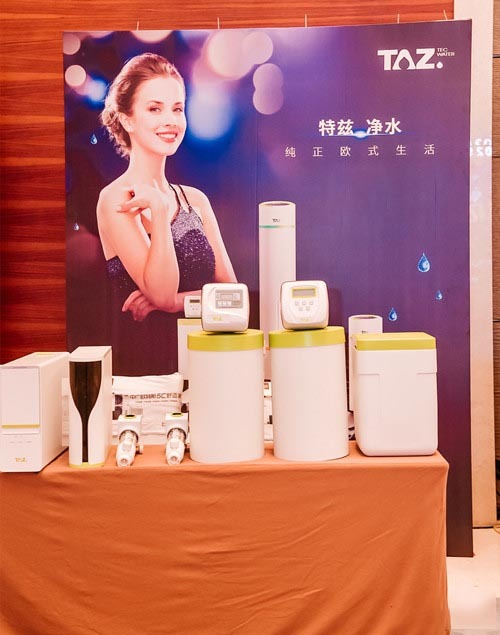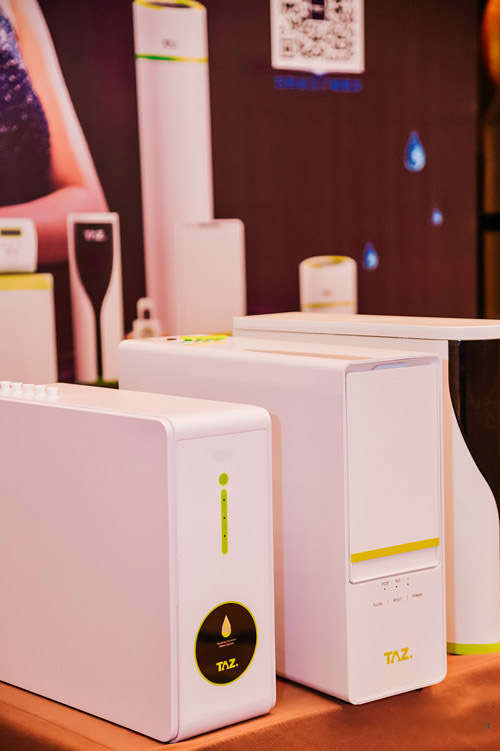|

# “特兹”荣获2020中国健康环境电器产业峰会质量诚信领军品牌

在净水界,也有个低调沉稳的品牌,专注研发电气化水处理系统及膜过滤技术并受到了德国当地政府和行业的认可,它就是TAZ特兹净水。

11月27日,由慧聪网主办的2020中国健康环境电器产业峰会暨品牌盛会颁奖盛典在深圳大梅沙京基洲际度假酒店隆重举行,来自全国各地的100+头部企业领袖、技术大咖、行业专家,以及来自全国各地近200名优质经销代理商共同见证了此次盛会。“特兹”荣获2020中国健康环境电器产业峰会质量诚信领军品牌奖

慧聪品牌盛会自9月启动报名以来,经过近3个月的激烈角逐,“特兹”最终竞得慧聪网2020中国健康环境电器产业峰会“质量诚信领军品牌”殊荣。

质量诚信是消费者对产品及品牌的认知评价,是对企业及服务的信任开端。质量是企业的生命,是企业的灵魂,任何一个企业要生存要发展就务必要提高产品质量,不断创新和超越,追求更高的目标。而在企业中,诚信代表着一种企业精神、企业文化和企业形象,是企业重要的无形资产,是企业在激烈竞争中生存下去的根本。质量诚信,是企业的核心竞争力,恪守质量,建设诚信,赢得一个良好的市场口碑,得到社会的认可,是企业成功的关键。特兹(上海)智能家居有限公司经公众投票和综合评定后,最终获得“慧聪网2020中国健康环境电器产业峰会质量诚信领军品牌”荣誉,这是企业在品牌之路上奋力续写的辉煌篇章。

作为业界一直备受瞩目的行业盛会,2020中国健康环境电器产业峰会,以“破局重构·蓬勃新生”为主题,以树立品牌榜样、表彰行业优秀为宗旨,打造一个权威专业、公平公正的展示平台,通过表彰为中国健康环境电器行业品牌发展作出重大贡献的企业和个人,弘扬行业品牌精神,提升企业品牌价值,用实际行动诠释品牌的力量,引领行业前进步伐。

在27日上午举办的产业峰会主题演讲中,特兹(上海)智能家居有限公司营销总监张达道为大家带来了《全屋净水:后疫情时代下健康投资新趋势》的精彩分享,展现了德国制造高端净水进军中国,抢滩全屋净水蓝海市场的信心与决心。特兹(上海)智能家居有限公司营销总监张达道演讲

TAZ特兹用德国匠人姿态,抢滩蓝海市场

相较于国外成熟的全屋净水市场,中国全屋净水市场还处于初步认知阶段,产品高度分散,缺乏能够专业领导市场品牌。

TAZ1979年诞生于德国的一个美丽小镇Carlsberg,一直走在德国家用净水技术的最前沿,依托公司雄厚且低调的科技背景,将独特的电气化水处理系统和成熟的膜过滤技术完美结合,成功打造了电气化全屋净水系统产品线,从工业净水领域进入并升级家用净水系统,同时整合创新的智能和物联网技术,为家庭净水带去福音。

自1979年成立至今,40多年来TAZ特兹一直是德国水处理行业的引领者。TAZ在德国拥有丰富的市政、家庭水处理经验,并能为用户提供最优质的用水解决方案。TAZ提供具有现代技术的先进水处理技术,最具代表的是商用反渗透系统PhoenixCompounding技术和离子交换型的软水机技术,TAZ可提供高端舒适的全屋用水解决方案。TAZ净水目标群体:新中产、高净值人群

对于中国消费者来说,高端全屋净水正在走进我们的每一个家庭。当高端市场在快速发展,追求更有美感、更高品质、更具创新力的产品成为中国的消费升级趋势。

作为高端净水品牌,TAZ的目标群体主要分为两类,一类是70后高净值人群,他们是消费市场的中坚力量,他们有高薪职业,有较高的社会地位,低调、理性、睿智,注重健康、教育、房产、金融类投资,不随波逐流,有自己的消费观念,愿意为有价值的产品付出。

另一类是80、90后中产人群,他们受过良好的教育,较多经历过海外留学,开始成为职场的中流砥柱。他们有品位,有格调,有国际化视野,对生活充满乐观向上的态度,成家立业、初为父母,懂得家人健康的可贵。

对于这类人群而言,中高端商场、时尚餐厅、咖啡馆、SPA等渠道不再是简单的交易场所,更是代表品质舒适的高端生活方式体验。TAZ看中的就是这一片高端市场的无限潜力。TAZ全屋净水系统360°品味纯正德系生活

TAZ特兹在深耕中国净水市场的过程中,坚守德国制造,凭借专业水净化科技与创新精神,凝聚水处理行业前沿技术,为中国消费者量身打造了TAZ系列和JUDO系列两大热门净水产品,拥有领先水处理反冲洗技术,全触控智能控制阀,成熟直饮水过滤膜等技术,为不同的家居生活提供专业RO反渗透解决方案。其中JUDO系列软水机还拥有QUICKSOFT-P专利软化技术,核心零部件均达到国际权威组织标准,掌握净水核心科技的TAZ特兹无疑是德式科技和严谨所淬炼出来的优质品牌。

此外,特兹24小时在线的优质售后服务让消费者尊享量身定做的水家装服务,安心无忧,这对于以“品质”立足的高端净水来说也至关重要。

2020年德国TAZ品牌正式进入中国,迅速打通全国经销商网络,目标覆盖全国30多个城市,2年内实现全国门店100家。

TAZ净水用德国匠人之心,一步一个脚印,做好品牌建设,用扎实的产品优势,为中国家庭带来更高品质的家居生活体验,用领先科技塑造挚爱生活。

`声明：本文由入驻焦点开放平台的作者撰写，除焦点官方账号外，观点仅代表作者本人，不代表焦点立场错误信息举报电话： 400-099-0099，邮箱：jubao@vip.sohu.com，或点此进行意见反馈，或点此进行举报投诉。`A B C D E F G H J K L M N P Q R S T W X Y Z
A - B - C - D - E
• A
• 鞍山
• 安庆
• 安阳
• 安顺
• 安康
• 澳门
• B
• 北京
• 保定
• 包头
• 巴彦淖尔
• 本溪
• 蚌埠
• 亳州
• 滨州
• 北海
• 百色
• 巴中
• 毕节
• 保山
• 宝鸡
• 白银
• 巴州
• C
• 承德
• 沧州
• 长治
• 赤峰
• 朝阳
• 长春
• 常州
• 滁州
• 池州
• 长沙
• 常德
• 郴州
• 潮州
• 崇左
• 重庆
• 成都
• 楚雄
• 昌都
• 慈溪
• 常熟
• D
• 大同
• 大连
• 丹东
• 大庆
• 东营
• 德州
• 东莞
• 德阳
• 达州
• 大理
• 德宏
• 定西
• 儋州
• 东平
• E
• 鄂尔多斯
• 鄂州
• 恩施
F - G - H - I - J
• F
• 抚顺
• 阜新
• 阜阳
• 福州
• 抚州
• 佛山
• 防城港
• G
• 赣州
• 广州
• 桂林
• 贵港
• 广元
• 广安
• 贵阳
• 固原
• H
• 邯郸
• 衡水
• 呼和浩特
• 呼伦贝尔
• 葫芦岛
• 哈尔滨
• 黑河
• 淮安
• 杭州
• 湖州
• 合肥
• 淮南
• 淮北
• 黄山
• 菏泽
• 鹤壁
• 黄石
• 黄冈
• 衡阳
• 怀化
• 惠州
• 河源
• 贺州
• 河池
• 海口
• 红河
• 汉中
• 海东
• 怀来
• I
• J
• 晋中
• 锦州
• 吉林
• 鸡西
• 佳木斯
• 嘉兴
• 金华
• 景德镇
• 九江
• 吉安
• 济南
• 济宁
• 焦作
• 荆门
• 荆州
• 江门
• 揭阳
• 金昌
• 酒泉
• 嘉峪关
K - L - M - N - P
• K
• 开封
• 昆明
• 昆山
• L
• 廊坊
• 临汾
• 辽阳
• 连云港
• 丽水
• 六安
• 龙岩
• 莱芜
• 临沂
• 聊城
• 洛阳
• 漯河
• 娄底
• 柳州
• 来宾
• 泸州
• 乐山
• 六盘水
• 丽江
• 临沧
• 拉萨
• 林芝
• 兰州
• 陇南
• M
• 牡丹江
• 马鞍山
• 茂名
• 梅州
• 绵阳
• 眉山
• N
• 南京
• 南通
• 宁波
• 南平
• 宁德
• 南昌
• 南阳
• 南宁
• 内江
• 南充
• P
• 盘锦
• 莆田
• 平顶山
• 濮阳
• 攀枝花
• 普洱
• 平凉
Q - R - S - T - W
• Q
• 秦皇岛
• 齐齐哈尔
• 衢州
• 泉州
• 青岛
• 清远
• 钦州
• 黔南
• 曲靖
• 庆阳
• R
• 日照
• 日喀则
• S
• 石家庄
• 沈阳
• 双鸭山
• 绥化
• 上海
• 苏州
• 宿迁
• 绍兴
• 宿州
• 三明
• 上饶
• 三门峡
• 商丘
• 十堰
• 随州
• 邵阳
• 韶关
• 深圳
• 汕头
• 汕尾
• 三亚
• 三沙
• 遂宁
• 山南
• 商洛
• 石嘴山
• T
• 天津
• 唐山
• 太原
• 通辽
• 铁岭
• 泰州
• 台州
• 铜陵
• 泰安
• 铜仁
• 铜川
• 天水
• 天门
• W
• 乌海
• 乌兰察布
• 无锡
• 温州
• 芜湖
• 潍坊
• 威海
• 武汉
• 梧州
• 渭南
• 武威
• 吴忠
• 乌鲁木齐
X - Y - Z
• X
• 邢台
• 徐州
• 宣城
• 厦门
• 新乡
• 许昌
• 信阳
• 襄阳
• 孝感
• 咸宁
• 湘潭
• 湘西
• 西双版纳
• 西安
• 咸阳
• 西宁
• 仙桃
• 西昌
• Y
• 运城
• 营口
• 盐城
• 扬州
• 鹰潭
• 宜春
• 烟台
• 宜昌
• 岳阳
• 益阳
• 永州
• 阳江
• 云浮
• 玉林
• 宜宾
• 雅安
• 玉溪
• 延安
• 榆林
• 银川
• Z
• 张家口
• 镇江
• 舟山
• 漳州
• 淄博
• 枣庄
• 郑州
• 周口
• 驻马店
• 株洲
• 张家界
• 珠海
• 湛江
• 肇庆
• 中山
• 自贡
• 资阳
• 遵义
• 昭通
• 张掖
• 中卫

1室1厅1厨1卫1阳台

1
2
3
4
5

0
1
2

1

1

0
1
2
3报名成功，资料已提交审核A B C D E F G H J K L M N P Q R S T W X Y Z
A - B - C - D - E
• A
• 鞍山
• 安庆
• 安阳
• 安顺
• 安康
• 澳门
• B
• 北京
• 保定
• 包头
• 巴彦淖尔
• 本溪
• 蚌埠
• 亳州
• 滨州
• 北海
• 百色
• 巴中
• 毕节
• 保山
• 宝鸡
• 白银
• 巴州
• C
• 承德
• 沧州
• 长治
• 赤峰
• 朝阳
• 长春
• 常州
• 滁州
• 池州
• 长沙
• 常德
• 郴州
• 潮州
• 崇左
• 重庆
• 成都
• 楚雄
• 昌都
• 慈溪
• 常熟
• D
• 大同
• 大连
• 丹东
• 大庆
• 东营
• 德州
• 东莞
• 德阳
• 达州
• 大理
• 德宏
• 定西
• 儋州
• 东平
• E
• 鄂尔多斯
• 鄂州
• 恩施
F - G - H - I - J
• F
• 抚顺
• 阜新
• 阜阳
• 福州
• 抚州
• 佛山
• 防城港
• G
• 赣州
• 广州
• 桂林
• 贵港
• 广元
• 广安
• 贵阳
• 固原
• H
• 邯郸
• 衡水
• 呼和浩特
• 呼伦贝尔
• 葫芦岛
• 哈尔滨
• 黑河
• 淮安
• 杭州
• 湖州
• 合肥
• 淮南
• 淮北
• 黄山
• 菏泽
• 鹤壁
• 黄石
• 黄冈
• 衡阳
• 怀化
• 惠州
• 河源
• 贺州
• 河池
• 海口
• 红河
• 汉中
• 海东
• 怀来
• I
• J
• 晋中
• 锦州
• 吉林
• 鸡西
• 佳木斯
• 嘉兴
• 金华
• 景德镇
• 九江
• 吉安
• 济南
• 济宁
• 焦作
• 荆门
• 荆州
• 江门
• 揭阳
• 金昌
• 酒泉
• 嘉峪关
K - L - M - N - P
• K
• 开封
• 昆明
• 昆山
• L
• 廊坊
• 临汾
• 辽阳
• 连云港
• 丽水
• 六安
• 龙岩
• 莱芜
• 临沂
• 聊城
• 洛阳
• 漯河
• 娄底
• 柳州
• 来宾
• 泸州
• 乐山
• 六盘水
• 丽江
• 临沧
• 拉萨
• 林芝
• 兰州
• 陇南
• M
• 牡丹江
• 马鞍山
• 茂名
• 梅州
• 绵阳
• 眉山
• N
• 南京
• 南通
• 宁波
• 南平
• 宁德
• 南昌
• 南阳
• 南宁
• 内江
• 南充
• P
• 盘锦
• 莆田
• 平顶山
• 濮阳
• 攀枝花
• 普洱
• 平凉
Q - R - S - T - W
• Q
• 秦皇岛
• 齐齐哈尔
• 衢州
• 泉州
• 青岛
• 清远
• 钦州
• 黔南
• 曲靖
• 庆阳
• R
• 日照
• 日喀则
• S
• 石家庄
• 沈阳
• 双鸭山
• 绥化
• 上海
• 苏州
• 宿迁
• 绍兴
• 宿州
• 三明
• 上饶
• 三门峡
• 商丘
• 十堰
• 随州
• 邵阳
• 韶关
• 深圳
• 汕头
• 汕尾
• 三亚
• 三沙
• 遂宁
• 山南
• 商洛
• 石嘴山
• T
• 天津
• 唐山
• 太原
• 通辽
• 铁岭
• 泰州
• 台州
• 铜陵
• 泰安
• 铜仁
• 铜川
• 天水
• 天门
• W
• 乌海
• 乌兰察布
• 无锡
• 温州
• 芜湖
• 潍坊
• 威海
• 武汉
• 梧州
• 渭南
• 武威
• 吴忠
• 乌鲁木齐
X - Y - Z
• X
• 邢台
• 徐州
• 宣城
• 厦门
• 新乡
• 许昌
• 信阳
• 襄阳
• 孝感
• 咸宁
• 湘潭
• 湘西
• 西双版纳
• 西安
• 咸阳
• 西宁
• 仙桃
• 西昌
• Y
• 运城
• 营口
• 盐城
• 扬州
• 鹰潭
• 宜春
• 烟台
• 宜昌
• 岳阳
• 益阳
• 永州
• 阳江
• 云浮
• 玉林
• 宜宾
• 雅安
• 玉溪
• 延安
• 榆林
• 银川
• Z
• 张家口
• 镇江
• 舟山
• 漳州
• 淄博
• 枣庄
• 郑州
• 周口
• 驻马店
• 株洲
• 张家界
• 珠海
• 湛江
• 肇庆
• 中山
• 自贡
• 资阳
• 遵义
• 昭通
• 张掖
• 中卫• 手机• 分享
• 设计
免费设计
• 计算器
装修计算器
• 入驻
合作入驻
• 联系
联系我们
• 置顶
返回顶部